# Program to check Happy Number Program in Java

In this tutorial you will learn about the Program to check Happy Number Program in Java and its application with practical example.

In this tutorial, we will learn to create a Java Program to check Happy Number Program in Java

## Prerequisites

Before starting with this tutorial we assume that you are best aware of the following Java programming topics:

• Java Operators.
• Basic Input and Output function in Java.
• Class and Object in Java.
• Basic Java programming.
• If-else statements in Java.
• Function in java.

## What is a happy number ?

A number is called a Happy Number when we replace the number by the sum of the squares of its digits, and repeat the process until the number reach to =”1″.

## Program to check Happy Number Program in Java

In this program we would find the given number is a Happy number or not , first of all we will take the input from the user and check whether it is Happy number or not. If it’s not then the program will show the output as it’s not a happy number.

## Output

Happy Number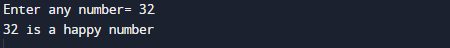Not a Happy Number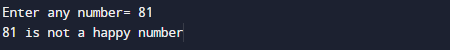In the above program, we have first declared and initialized a set variables required in the program.

• num,number= it will hold entered number.
• temp = it will hold temp value
• sum= for adding digit sum.
• rem=  it will hold remainder of numbers.

After Declaring variables we will take a number form user to find given number is a happy number or not.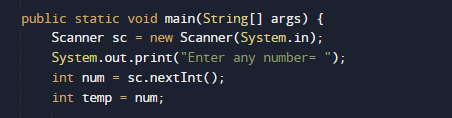after taking values we will check given number is valid number or not.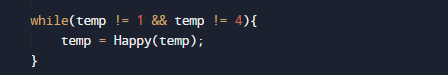and the temp value to function Happy() where we iterate the number to its last value.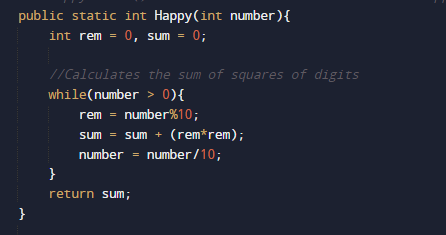the function return the square of each digit present in number and finally at last we will check if the sum is equal to 1 it is a Happy number.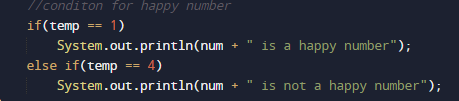if it is not equal to “1” than it is not a happy number.

In this tutorial we have learn about the Program to check Happy Number Program in Java and its application with practical example. I hope you will like this tutorial.